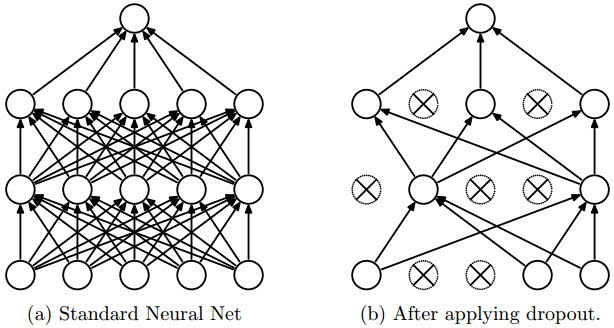Categories:

Updated:

# Diagnosing and Solving Bias/Variance

## Diagnosing

High train set error -> High bias

If dev set error is much higher than train set error -> High variance

## Solving

### High bias

• bigger network
• train more epochs

### High Variance

• more data
• regularization

# Regularization Techniques

## DropoutDropout is the common regularization technique in deep learning.

We set hyperparameter $p$, typically as a value between 0 and 0.5.

Then during training, we deactivate random (number of units)$\times p$ units on a particular layer.

This improve generalization because it forces your layer to learn with different units the same concept.

Number of our units on a particular layer has decreased, so we multiply $\frac{1}{1-p}$ to units of that layer to compensate.

## L2(Frobenius) Regularization

L2 regularization is the common regularization technizue in machine learning.

Frobenius Norm

Then, term $\frac{\lambda}{m}W^{[l]}$ is added to our original $dW^{[l]}$

## L1 Regularization

You don’t use L1 regularization much in practice

L1 Norm

The rest is same as L2 regularization.

### Why does L2 and L1 regularization prevent overfitting?

Regularization decays weight(makes weight close to 0), thus restrains the impact of each units.

Also, it makes $z$ close to 0. Since part of tanh or sigmoid function near 0 is similar to a linear function, it reduces complexity.

# Other ways to reduce variance

### Data Augumentation

In a image recognition task, flipping or rotating image and adding them to training set will reduce variance.

### Early Stopping

training for less epochs might reduce dev set error. However, Andrew Ng doesn’t recommend early stopping. He says that if you do early stopping, training set error is not sufficiently low thus problem of high bias rises. He recommends addressing high variance and high bias seperately, not sacrificing one to get another.

Categories: